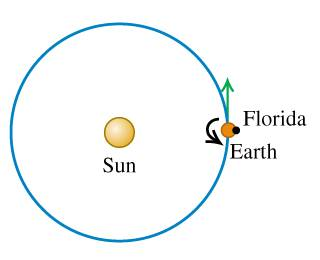# Spacecraft launched from the earth

xyd
1. A spacecraft is to be launched from the surface of the earth so that it will escape from the solar system altogether.

Find the speed relative to the center of the earth with which the spacecraft must be launched. Take into consideration the gravitational effects of both the earth and the sun, and include the effects of the earth's orbital speed, but ignore air resistance.Assume that the direction of launch is the direction of the earth’s motion in its orbit.## Homework Equations

vescape=$$\sqrt{\frac{2 G M}{R}}$$

3. The Attempt at a Solution :
11858 m/s
12321 m/s
11200 m/s

Gold Member
no attempt, no help!!! proceed and try something, and show us your work. if you are stuck, we are here to help.

xyd
First, I am sorry that I did not show my attempt clearly. This is my first time to post a homework thread. Sorry again!

And then here are my attempt.

First of all, I take:
mass of Sun: M = 1.99 x 1030 kg
mass of Earth: m = 5.97 x 1024 kg
orbit radius of the Earth: 1.50 x 1011 m

Then, suppose the Earth is in circular motion, we get the speed of the earth relative to the mass point of the Sun:
VEarth = 29747 m/s

As well as the escape speed:
Vescape = $$\sqrt{2}$$ VEarth = 42069 m/s

Since the question asks for the speed relative to the earth, I just subtract them:
Vescape - VEarth = 12322 m/s

This is one of my attempt.

Another attempt was that I also consider the rotation effect of Earth, but it was also wrong.

One more attempt was I calculate the escape speed of Earth directly using the escape speed equation above... but it was wrong again.

xyd

Mentor
You need to escape both the Earth and the Sun. I think you'll find that the velocity to escape the Sun is much larger than Earth escape velocity.

Concerning the possibility of velocity assist from Earth motion, consider that the Earth is not only traveling around the Sun, but rotating on its axis...

xyd
Well... yes. Actually, I tried it once, but I still failed. However, I really found the velocity escape from Sun is really much larger. Hence, could I make such a conclusion:
the velocity of the ship relative to the Sun is the escape velocity from the Sun??

Whatever, thanks a lot!

Mentor
Perhaps you should think in terms of the work that needs to be done in order to remove the spacecraft from the Earth's surface and then from the Sun at the distance of Earth's orbit. Basically, it's a potential problem. Once you have the total work required, you have the necessary kinetic energy (velocity). Subtract any "helpful boosts" that you get from Earth motions.

xyd
Yes, it makes sense. I would try this way. Thank you.

In addition, I believe the rotation of the Earth should not be considered as what the question mentioned
"Take into consideration the gravitational effects of both the earth and the sun, and include the effects of the earth's orbital speed" (I do not know how to quote a sentence...)

Then, the "helpful boost" could only be gravitation of both the earth and sun, and the earth's orbital now, right?

xyd
Could I do the question this way:

Suppose:
- Ks is the minimum kinetic energy required to escape (relative to the mass center of the sun);
- Ke is the minimum kinetic energy required to escape (relative to the mass center of the earth);
- Ps is the potential energy from the sun
- Pe is the potential energy from the earth

Then, we have:
Ks + Ps + Pe = something

According to the conservation of energy, "something" remains the same during the travel.
Now let the ship travel to infinite distance from the sun. Then the Ke must be zero; Ps and Pe should also be zero. Thus,
Ks + Ps + Pe = 0

Then we get Ks. Ke would also be solved after subtracting the kinetic energy of the earth (earth's orbital speed)

Gold Member
gravity of earth and sun are the 'unhelpful' boost. orbital velocity of earth is helpful.

use potential at infinity = 0 and find gravitational potential of spacecraft o earth due to both sun and earth, and its initial velocity is not 0 but the orbital velocity of earth. now solve.

xyd
One more question, is the kinetic energy 0 at infinity??

Gold Member
it may not be so. its not about energy, its about potential, not potential energy

Mentor
One more question, is the kinetic energy 0 at infinity??

If the launch velocity is exactly the required amount to "escape", then yes, the KE at infinite distance would be zero (that is, asymptotically it goes to zero).

xyd
Get it! Thank you.

xyd
OK, my answer has been proved right. I was quite nervous as it was already the final attempt. Thanks so much for your help, gneill and supratim1!!

mistergrinch
When I use simple energy conservation I get:

1/2v0^2 - G*M_s/r_s - G*M_e/r_e = 0 => v0 = 43.6 km/s

This is the speed relative to the sun required to escape both sun and earth gravity.

Since the earth is orbiting at 29.8 km/s, subtracting I get 13.8 km/s for the escape speed relative to earth if we launch in the direction of the earth's orbit.

This is not the correct answer according to Wikipedia! Can anyone tell me what I'm doing wrong here? Thanks.